# 原理化毛发BSDF¶Melanin concentration选项下的原理化毛发 BSDF节点界面。

Cycles Only

Tip

## 输入¶

Hint

$\sigma_{a} = \frac{\ln(Color)} {\left(5.969 - 0.215\beta_{N} + 2.532\beta_{N}^{2} - 10.73\beta_{N}^{3} + 5.574\beta_{N}^{4} + 0.245\beta_{N}^{5}\right)^{2}}$Hint

$melanin\_qty = -\ln(\max(1.0 - Melanin, 0.0001))$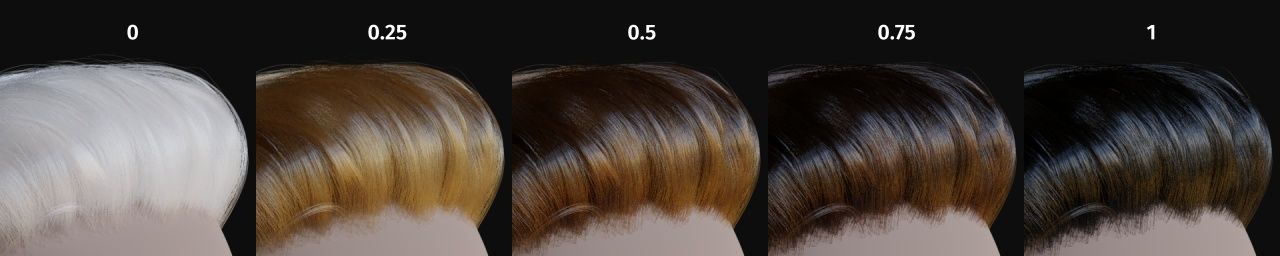Hint

$\begin{split}\sigma_{a} = eumelanin * \left[\begin{matrix} 0.506 \\ 0.841 \\ 1.653 \\ \end{matrix}\right] + pheomelanin * \left[\begin{matrix} 0.343 \\ 0.733 \\ 1.924 \\ \end{matrix}\right]\end{split}$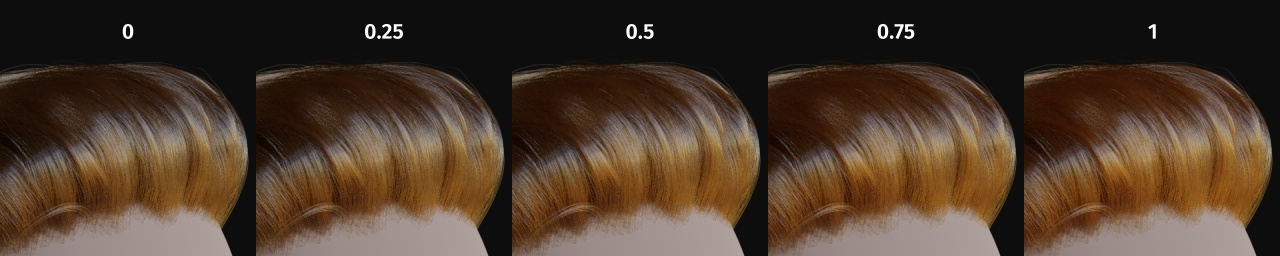Hint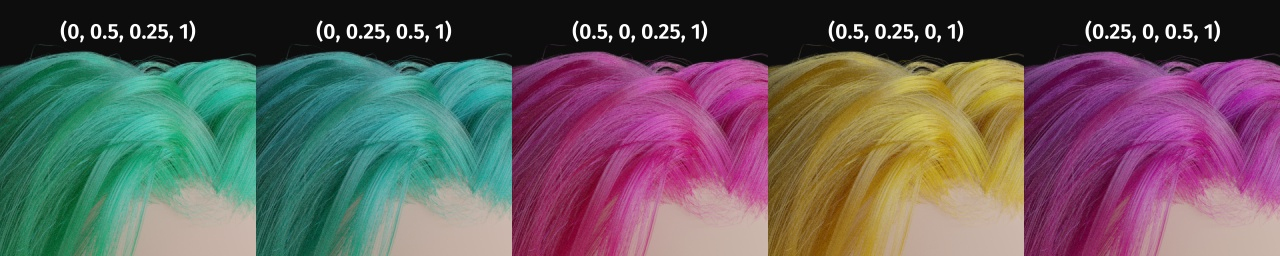Specify how much the glints are smoothed in the direction of the hair shaft. Too low values will smoothen the hair to the point of looking almost metallic, making glints look like Fireflies; while setting it too high will result in a Lambertian look.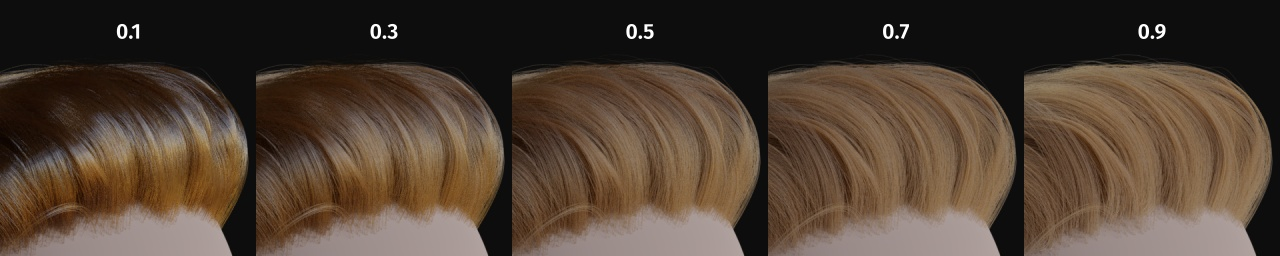Hint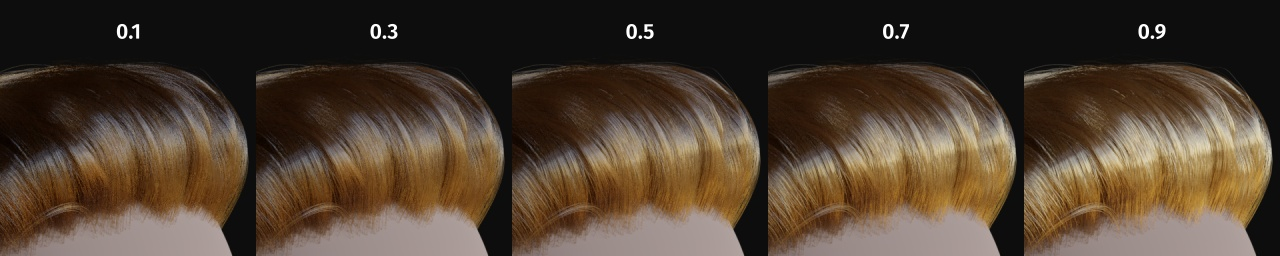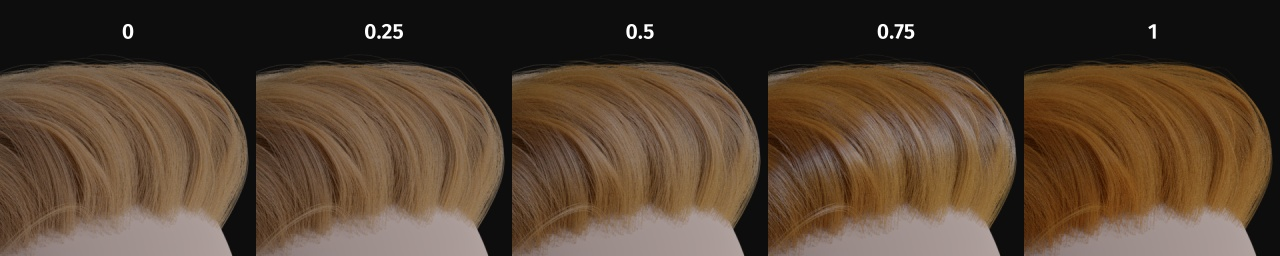IOR

Hint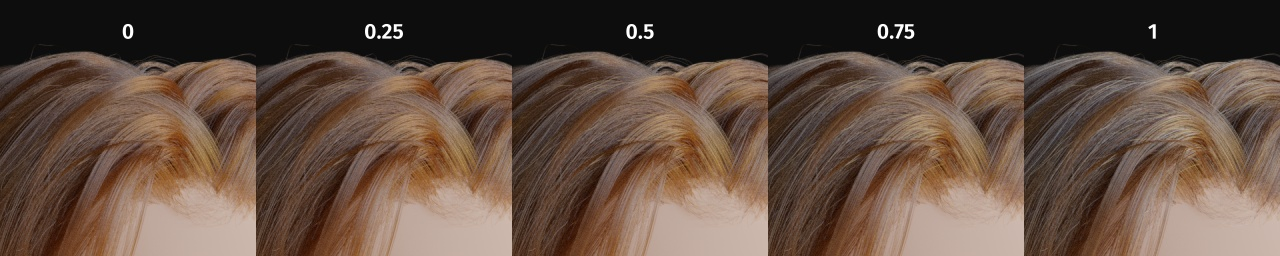Hint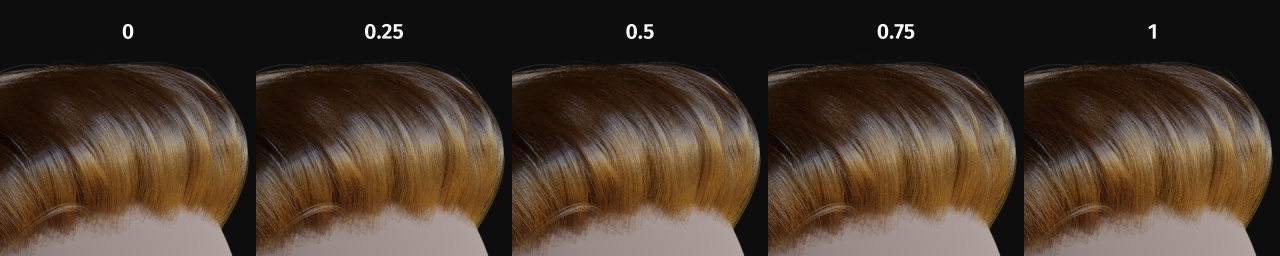## 属性¶

• White (Melanin $$0$$)

• 亚麻色 (黑色素值设为 $$0.25$$)

• 淡红色 (黑色素值设为 $$0.5$$)

• 棕色 (黑色素值设为 $$0.75$$)

• 黑色 (黑色素值设为 $$1$$)